# JP4649781B2 - Image processing method and apparatus - Google Patents

## Info

Publication number
JP4649781B2
JP4649781B2 JP2001187146A JP2001187146A JP4649781B2 JP 4649781 B2 JP4649781 B2 JP 4649781B2 JP 2001187146 A JP2001187146 A JP 2001187146A JP 2001187146 A JP2001187146 A JP 2001187146A JP 4649781 B2 JP4649781 B2 JP 4649781B2
Authority
JP
Japan
Prior art keywords
value
pixel
threshold
level
image
Prior art date
Legal status (The legal status is an assumption and is not a legal conclusion. Google has not performed a legal analysis and makes no representation as to the accuracy of the status listed.)
Expired - Fee Related
Application number
JP2001187146A
Other languages
Japanese (ja)
Other versions
JP2003008935A (en
JP2003008935A5 (en
Inventor

Original Assignee
ソニー株式会社
Priority date (The priority date is an assumption and is not a legal conclusion. Google has not performed a legal analysis and makes no representation as to the accuracy of the date listed.)
Filing date
Publication date
Application filed by ソニー株式会社 filed Critical ソニー株式会社
Priority to JP2001187146A priority Critical patent/JP4649781B2/en
Publication of JP2003008935A publication Critical patent/JP2003008935A/en
Publication of JP2003008935A5 publication Critical patent/JP2003008935A5/ja
Application granted granted Critical
Publication of JP4649781B2 publication Critical patent/JP4649781B2/en
Expired - Fee Related legal-status Critical Current
Anticipated expiration legal-status Critical

• 238000003672 processing method Methods 0.000 title claims description 14
• 238000006243 chemical reactions Methods 0.000 claims description 73
• 238000000034 methods Methods 0.000 claims description 21
• 238000001914 filtration Methods 0.000 claims description 13
• 230000001131 transforming Effects 0.000 claims description 8
• 230000001276 controlling effects Effects 0.000 claims description 6
• 238000005286 illumination Methods 0.000 abstract description 71
• 238000007906 compression Methods 0.000 abstract description 25
• 238000010586 diagrams Methods 0.000 description 22
• 238000007796 conventional methods Methods 0.000 description 10
• 230000000875 corresponding Effects 0.000 description 9
• 230000001965 increased Effects 0.000 description 8
• 230000005540 biological transmission Effects 0.000 description 6
• 230000000694 effects Effects 0.000 description 6
• 280000456776 Retinex companies 0.000 description 5
• 239000007787 solids Substances 0.000 description 5
• 241001442055 Vipera berus Species 0.000 description 4
• 230000001186 cumulative Effects 0.000 description 4
• 238000009499 grossing Methods 0.000 description 3
• 230000001629 suppression Effects 0.000 description 3
• 239000000470 constituents Substances 0.000 description 2
• 230000037094 Cmin Effects 0.000 description 1
• 230000004069 differentiation Effects 0.000 description 1
• 238000000605 extraction Methods 0.000 description 1
• 238000010606 normalization Methods 0.000 description 1
• 230000002093 peripheral Effects 0.000 description 1
• 230000002441 reversible Effects 0.000 description 1

## Images

•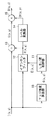•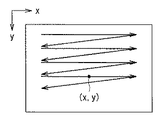•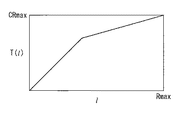•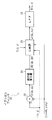•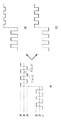•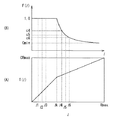•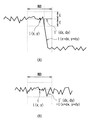•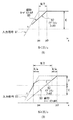•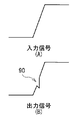•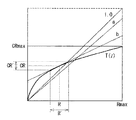•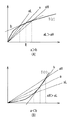•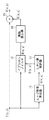•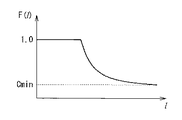••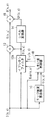•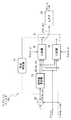•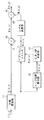•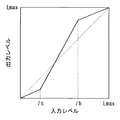•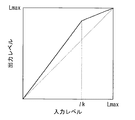••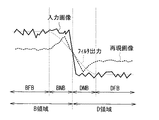## Classifications

• GPHYSICS
• G06COMPUTING; CALCULATING; COUNTING
• G06TIMAGE DATA PROCESSING OR GENERATION, IN GENERAL
• G06T5/00Image enhancement or restoration
• G06T5/20Image enhancement or restoration by the use of local operators
• GPHYSICS
• G06COMPUTING; CALCULATING; COUNTING
• G06TIMAGE DATA PROCESSING OR GENERATION, IN GENERAL
• G06T5/00Image enhancement or restoration
• G06T5/001Image restoration
• G06T5/002Denoising; Smoothing
• GPHYSICS
• G06COMPUTING; CALCULATING; COUNTING
• G06TIMAGE DATA PROCESSING OR GENERATION, IN GENERAL
• G06T7/00Image analysis
• G06T7/10Segmentation; Edge detection
• G06T7/12Edge-based segmentation
• GPHYSICS
• G06COMPUTING; CALCULATING; COUNTING
• G06TIMAGE DATA PROCESSING OR GENERATION, IN GENERAL
• G06T2207/00Indexing scheme for image analysis or image enhancement
• G06T2207/10Image acquisition modality
• G06T2207/10016Video; Image sequence
• GPHYSICS
• G06COMPUTING; CALCULATING; COUNTING
• G06TIMAGE DATA PROCESSING OR GENERATION, IN GENERAL
• G06T2207/00Indexing scheme for image analysis or image enhancement
• G06T2207/20Special algorithmic details
• GPHYSICS
• G06COMPUTING; CALCULATING; COUNTING
• G06TIMAGE DATA PROCESSING OR GENERATION, IN GENERAL
• G06T2207/00Indexing scheme for image analysis or image enhancement
• G06T2207/20Special algorithmic details
• G06T2207/20172Image enhancement details
• G06T2207/20192Edge enhancement; Edge preservation

## Abstract

By the use of an epsilon filter, in the case where a plurality of different illuminations exist, a boundary between the illuminations can be appropriately extracted, and an unnatural image pattern can be prevented from being generated, therefore subjectively preferable compression of a dynamic range can be achieved. An edge strength G(x, y) is calculated per position on an input image, and a threshold E(x, y) of an epsilon filter ( 12 ) is controlled on the basis of the edge strength G(x, y). The epsilon filter ( 12 ) filters the input image on the basis of the controlled threshold E(x, y). On the basis of the edge strength G(x, y), the threshold E of the epsilon filter ( 12 ) is adaptively changed according to a local gradient of a pixel value I(x, y), so in the case of using a linear lowpass filter or a fixed threshold epsilon filter, an illumination boundary can be more accurately extracted.

## Description


BACKGROUND OF THE INVENTION
The present invention can be suitably used for various image input / output devices such as a television, a video tape recorder, a still camera, a video camera and a printer. The present invention relates to an image processing method and apparatus for reproducing in a narrow image apparatus.

[Prior art]
Conventionally, for example, for the conversion of the gradation characteristics of an image, a function having an input / output relationship as shown by a solid line in FIG. ) For conversion (hereinafter referred to as “level conversion”). In FIG. 18, the horizontal axis represents the pixel level (input level) 1 of the input image, and the vertical axis represents the pixel level (output level) T (l) of the output image obtained by level conversion processing. Lmax represents the maximum level that each pixel of the input / output image can take. The contrast of the image after level conversion increases as the slope of the level conversion function increases. In the example of FIG. 18, the slope of the straight line indicating the level conversion function between the high level with the input level lb as the boundary and the low level side with the input level ls as the boundary is the slope of the intermediate level (input levels ls to lb). It is smaller than Therefore, in the level conversion using the function shown in FIG. 18, the contrast at the intermediate level is increased by sacrificing the contrast at the high level and the low level.

The level conversion function is not limited to the one shown in FIG. 18, and for example, the one shown by the solid line in FIG. In the level conversion function shown in FIG. 19, the slope of the straight line on the high level side is smaller than the slopes at the low level and the intermediate level with the input level lk as a boundary. Accordingly, in the level conversion using the function shown in FIG. 19, the contrast at the low level and the intermediate level can be increased by sacrificing the contrast at the high level. Further, a more continuous level conversion function may be used as compared with the functions shown in FIGS. 18 and 19 such as the following gamma function of equation (1) and LOG function of equation (2). Note that g in the equation (1) is a parameter for adjusting the slope of the function.

[Expression 1]

[Expression 2]

As another conventional example, there is a method of adaptively changing the level conversion function in accordance with the frequency distribution of the pixel level of the input image. A typical example is a method called histogram equalization. FIGS. 20A and 20B show the principle of this histogram equalization. In FIG. 20A, the horizontal axis represents the pixel level (input level) 1 of the input image, and the vertical axis represents the frequency (frequency or cumulative frequency). Fmax is the maximum value of the cumulative frequency, and is the total number of pixels used for calculating the frequency. In this method, as shown in FIG. 20A, first, a frequency distribution H (l) relating to the pixel level 1 of the input image is generated, and then the cumulative frequency distribution C (l using the following equation (3): ) Is generated.

[Equation 3]

A level conversion function T (l) is generated by normalizing the vertical axis of the cumulative frequency distribution C (l) to a level range that can be taken by the output image using the following equation (4) (FIG. 20). (B)). By using this function T (l), it is possible to increase the contrast of a region (region having a large area) constituted by a level having a high appearance frequency.

[Expression 4]

When the input image is used in an environment with a smaller dynamic range, that is, with a small number of bits representing the pixel level (when transmitted through a transmission path with a small number of bits, when displayed on a display device, or a storage device) In the case of storing in (for example), it is necessary to compress the dynamic range. Conventionally, level conversion similar to the above-described method is also used for dynamic range compression processing for such a purpose. However, in this case, the maximum level of the output image of the level conversion function is a value smaller than that of the input image.

On the other hand, in the document “Z. Rahman, et, alt .:“ A Multiscale retinex for color rendition and dynamic range compression in Applications of Digital image Processing ”, XIX Proc. SPIE 2847 (1996), it changes slowly and spatially. A method of compressing the entire dynamic range by extracting a component of illumination light using a low-pass filter and compressing the component is proposed (hereinafter, this method is referred to as “Multiscale retinex method”). A narrow-band linear low-pass filter is used to extract the illumination component. In this method, as shown in the following formula (5), logarithmic values of the input pixel value I (x, y) and the low-pass filter output LPF (I (x, y)) are taken and the latter is subtracted from the former. As a result, the dynamic range is compressed.

[Equation 5]

[Problems to be solved by the invention]
By the way, in the conventional level conversion method described above, a level conversion function having a monotonically increasing property is used in order to avoid generation of an unnatural image. For this reason, when the contrast (gradient of the level conversion function) in any one of the level ranges is increased, there is a problem that the contrast is lowered in the other level ranges.

In addition, the Multiscale retinex method can reproduce images with higher contrast by sacrificing monotonic increase, but if the lighting conditions change rapidly, the linear filter can extract the change. There is a problem that noise that cannot be subjectively generated occurs.

For example, as shown in FIG. 21, when a linear low-pass filter is applied to an image (solid line in the figure) in which two regions having different illumination conditions are adjacent to each other, A blurred signal is obtained as the filter output. When this is regarded as an illumination component, in the left area (B area) of the illumination boundary, the vicinity of the boundary (BNB area) has a lower illumination level than the part far away from the boundary (BFB area). The above equation (5) is equivalent to dividing the input signal by the illumination component, and means that the larger the illumination component is, the larger the compression is. Therefore, as a result, the BNB region of the reproduced image (thick broken line in the figure) Overshoot occurs. Conversely, in the area on the right side of the illumination boundary (D area), the vicinity of the boundary (DNB area) is considered to have a higher illumination level than the portion (DFB) far from the boundary, and undershoot occurs. To avoid this problem, the Multiscale retinex method uses multiple linear low-pass filters with different scales, and uses a method of combining the results obtained by each low-pass filter with a linear load, but the weight for each scale is fixed. The above problems are not sufficiently suppressed.

Therefore, it is conceivable to use a non-linear filter such as an epsilon filter instead of a linear low-pass filter for extraction of illumination components. The epsilon filter has an excellent ability to preserve edges compared to a linear filter, and can extract illumination components more effectively even in an image in which different illumination light exists. However, the fixed threshold epsilon filter, which is generally used for the purpose of noise removal, forms a discontinuous waveform in the vicinity of the edge at the output, so this was used for dynamic range compression. In this case, an unnatural image pattern that does not exist in the original image may occur in the reproduced image after compression.

The present invention has been made in view of such problems, and an object of the present invention is to use an epsilon filter to appropriately extract the boundaries even when there are a plurality of different illuminations. It is an object of the present invention to provide an image processing method and apparatus capable of suppressing the occurrence of a complex image pattern and realizing a subjectively preferable dynamic range compression.

[Means for Solving the Problems]
An image processing method according to the present invention includes an edge intensity calculation process for calculating edge intensity for each position on an input image, and a threshold control process for controlling a threshold value of an epsilon filter based on the calculated edge intensity. And a filtering process in which the input image is filtered by an epsilon filter using the threshold value controlled in the threshold control process, and a coefficient for converting the pixel value according to the output value of the filtering process. And a pixel value conversion process in which pixel values are converted for each pixel based on the calculated coefficients.

An image processing apparatus according to the present invention includes an edge strength calculation unit that calculates edge strength for each position on an input image, an epsilon filter that performs filtering on the input image using a set threshold, and edge strength. Based on the edge intensity calculated by the calculation means, a threshold control means for controlling the threshold value used in the epsilon filter, and a coefficient for converting the pixel value according to the output value from the epsilon filter are calculated. The pixel value conversion means for converting the pixel value for each pixel by the calculated coefficient is provided.

In the image processing method and apparatus according to the present invention, the edge strength is calculated for each position on the input image, and the threshold value used in the epsilon filter is controlled based on the calculated edge strength. Then, a coefficient for converting the pixel value is calculated according to the output value from the epsilon filter, and the pixel value is converted for each pixel by the calculated coefficient. As a result, even when there are a plurality of different illuminations, it is possible to appropriately extract the boundaries in the filter processing by the epsilon filter.

Also, In the image processing method and apparatus according to the present invention, Calculating two thresholds with different sizes and controlling the threshold Yeah. At this time, the epsilon filter , Filter processing is performed using different threshold values depending on whether the value of the neighboring pixel is larger or smaller than the target pixel. This And In the filter processing, the influence of the illumination level in the change of the pixel value is reduced, and the illumination component is extracted more appropriately.

DETAILED DESCRIPTION OF THE INVENTION
Hereinafter, embodiments of the present invention will be described in detail with reference to the drawings.

[First Embodiment]
First, an input image signal processed in the image processing apparatus according to the present embodiment will be described. The input image signal processed in this image processing apparatus is a signal of time-series pixel values obtained by scanning a two-dimensional digital image in the order of the horizontal direction and the vertical direction as shown in FIG. In the present embodiment, a pixel value corresponding to an arbitrary position (x, y) on the two-dimensional image is represented as I (x, y), and this is processed as an input image signal.

Next, the configuration of the image processing apparatus according to the present embodiment will be described. As shown in FIG. 1, the image processing apparatus includes an edge intensity calculator 10, a threshold controller 11, an epsilon filter 12, a divider 13, a level converter 14, and a multiplier 15. I have.

The edge strength calculator 10 has a function of calculating the edge strength G (x, y) of the pixel value I (x, y) at each position of the input image. As the edge strength G (x, y), for example, a primary differential value of I (x, y) as given by the following equation (6) can be used.

[Formula 6]

Alternatively, a value according to the following equation (7) having a smoothing effect to suppress the influence of noise can be used as the edge strength G (x, y).

[Expression 7]

Here, in the equations (6) and (7), d is a constant indicating a minute distance for calculating the differentiation. The edge strength G (x, y) calculated by the edge strength calculator 10 is sent to the threshold controller 11.

Based on the edge strength G (x, y) calculated by the edge strength calculator 10, the threshold controller 11 determines the threshold E (x, y) used in the epsilon filter 12 described later for each pixel. It has a function to determine. Due to the function of the threshold controller 11, the threshold value E (x, y) is set to a smaller value as the edge strength G (x, y) is larger, for example, by using the following equation (8). Be controlled.

[Equation 8]

In equation (8), Gmin, Gmax, Emin, and Emax are constants for converting the edge strength G (x, y) to the threshold value E (x, y), and the minimum value and the maximum value of the edge strength, respectively Value, the minimum value and the maximum value of the threshold value E (x, y). The threshold value E (x, y) determined by the threshold value controller 11 is sent to the epsilon filter 12.

As shown in FIG. 4, the epsilon filter 12 includes, for example, a difference unit 20, an absolute value calculator 21, a comparator 22, and a linear low-pass filter (denoted as LPF in the drawing) 23. Has been. The epsilon filter 12 is a two-dimensional filter, and has a function of performing non-linear filtering on the input image using the threshold value E (x, y) determined by the threshold controller 11. Yes. The output R (x, y) of the epsilon filter 12 is sent to the divider 13 and the level converter 14 as an illumination component.

In order to remove the illumination component calculated by the epsilon filter 12 from the input image, the divider 13 converts each pixel value I (x, y) of the input image to the illumination component R as shown in the following equation (9). Divide by (x, y). The non-illumination component S (x, y) obtained as a result of the division is sent to the multiplier 15.

[Equation 9]

The level converter 14 compresses the illumination component R (x, y) calculated by the epsilon filter 12 by performing level conversion using a level conversion function T (l) as shown in the following equation (10): It has a function of calculating the corrected illumination component CR (x, y).

[Expression 10]

As the level conversion function T (l) used in the level converter 14, for example, a function as shown in FIG. 3 can be used. In FIG. 3, Rmax and CRmax represent the maximum values of the input level and the output level, respectively.

The multiplier 15 restores the image signal by adding the corrected illumination component CR (x, y) to the non-illumination component S (x, y) as shown in the following equation (11). . The image signal O (x, y) indicating the restored result is output to a transmission path, a storage device, a display device, or the like (not shown).

## EQU11 ##

In the present embodiment, the divider 13, the level converter 14 and the multiplier 15 correspond to a specific example of “pixel value converting means” in the present invention.

Next, the operation and operation of the image processing apparatus configured as described above will be described. The following description also serves as a description of the image processing method according to the present embodiment.

In this image processing apparatus, a signal indicating an input image is input to the edge intensity calculator 10, the epsilon filter 12 and the multiplier 13. First, the edge strength calculator 10 calculates the edge size, that is, the edge strength G (x, y) for each position of the input image. At this time, the edge strength calculator 10 uses, for example, the above-described equation (6) or (7) so that the larger the primary differential value of the pixel value in the region near the target pixel is, the larger the value is. Thus, the edge strength G (x, y) is calculated. The edge strength calculator 10 outputs the calculated edge strength G (x, y) to the threshold controller 11.

The threshold controller 11 controls the threshold E of the epsilon filter 12 based on the edge strength G (x, y). More specifically, the threshold value controller 11 determines the size of the threshold value E (x, y) for each pixel using, for example, the above equation (8), and the edge strength G (x, y). Control is performed so that the threshold value E (x, y) decreases as the value increases. The threshold controller 11 outputs the determined threshold value E (x, y) to the epsilon filter 12.

The epsilon filter 12 performs a filtering process on the input image using the threshold value E (x, y) determined by the threshold value controller 11.

More specifically, the filtering process in the epsilon filter 12 is performed as follows, for example, with the configuration shown in FIG. In the epsilon filter 12, as shown in FIG. 4, the subtractor 20 includes a signal indicating the current pixel value I (x, y) and the pixel value I (x + dx, y + dy) in the neighboring region NB. Is input. The difference unit 20 calculates a difference between the value I (x, y) of the target pixel and the value I (x + dx, y + dy) of the pixel in the neighboring area NB. The difference unit 20 sequentially calculates the difference value for all the pixels in the neighboring area NB, and outputs the value D (dx, dy) to the absolute value calculator 21 in association with each neighboring pixel.

The absolute value calculator 21 calculates the absolute value AD (dx, dy) of each difference value D (dx, dy) sent from the differentiator 20. The absolute value calculator 21 outputs the calculated absolute value AD (dx, dy) to the comparator 22.

The comparator 22 receives the absolute value AD (dx, dy) calculated by the absolute value calculator 21 and signals indicating the value I (x, y) of the target pixel and the pixels in the neighboring region NB. A signal indicating the value I (x + dx, y + dy) of the signal and a threshold value E (x, y) determined by the threshold controller 11 are input. The comparator 22 compares the absolute value AD (dx, dy) and the threshold value E (x, y) as shown in the following expression (12), and according to the result, the value I (( Either x, y) or the neighboring pixel value I (x + dx, y + dy) is selected and output to the linear low-pass filter 23 as a value J (dx, dy).

[Expression 12]

In the linear low-pass filter 23, when the values J (dx, dy) corresponding to all the pixels in the neighboring region NB are calculated by the comparator 22, the weighted average value R (x, y) according to the following equation (13) is used. ) Is calculated.

[Formula 13]

Here, NB is a set of relative coordinates that define a neighboring region in the filtering process. Further, a (dx, dy) is a weighting factor for each pixel value. As this linear low-pass filter 23, for example, an average value filter as shown in the following equation (14) can be used.

[Expression 14]

In the equation (14), N represents the number of pixels in the near region NB. The purpose of the epsilon filter 12 is to remove fine structures in the image and to extract a grouped region, and it is desirable that the neighborhood region is large.

The value R (x, y) obtained by the epsilon filter 12 as described above is considered to approximately represent the illumination component included in the image. The epsilon filter 12 outputs the value R (x, y) as an illumination component to the divider 13 and the level converter 14.

The divider 13 divides each pixel value I (x, y) of the input image by the illumination component R (x, y) as shown in the above equation (9), thereby epsilon filter 12 from the input image. The non-illumination component S (x, y) obtained as a result is output to the multiplier 15.

On the other hand, the level converter 14 compresses the illumination component R (x, y) calculated by the epsilon filter 12 by performing level conversion using, for example, a level conversion function T (l) as shown in FIG. The component CR (x, y) is calculated. The level converter 14 outputs the calculated corrected illumination component CR (x, y) to the multiplier 15.

In the multiplier 15, the image signal is restored by integrating the non-illumination component S (x, y) output from the divider 13 with the corrected illumination component CR (x, y) output from the level converter 14. To do. Here, considering the overall calculation in the above-described divider 13, level converter 14 and multiplier 15, it is possible to add the corrected illumination component CR (x, y) to the non-illumination component S (x, y). As shown in the equation (16) described later, a coefficient F (R (x, y)) for converting the pixel value according to the output value R (x, y) from the epsilon filter 12 is calculated. Is integrated with the corresponding input pixel value I (x, y), which corresponds to the conversion of the pixel value for each pixel to compress the dynamic range.

As described above, the image signal O (x, y) output from the multiplier 15 is an image device having a relatively narrow dynamic range than the input image, that is, an environment in which the number of bits representing the pixel level is small (the number of bits). In the case of transmission on a transmission line with a small amount of data, when displaying on a display device, or when storing in a storage device).

Next, referring to FIG. 5 and FIG. 6, when the dynamic range is compressed using the epsilon filter 12 in the present embodiment, the compression is performed using the conventional method (the level conversion function having monotonically increasing property). The effectiveness with respect to the case of performing)

FIG. 5A is a diagram representing the pixel value I (x, y) of the input image and the output R (x, y) from the epsilon filter 12 in this embodiment as a one-dimensional signal. FIG. 5B shows a result (reproduced image) obtained by performing dynamic range compression on the input image shown in FIG. 5A by a conventional level conversion method, and FIG. 5C shows the present embodiment. The result of having performed the compression of the dynamic range in a form is shown.

6A and 6B show the relationship between the pixel level of each region, the level conversion function T (l), and the coefficient calculation function F (l). Here, the coefficient calculation function F (l) is defined as the following equation (15) using the level conversion function T (l).

[Expression 15]

Using this coefficient calculation function F (l) and considering the above-mentioned formulas (9) and (10), the formula (11) that gives the output image O (x, y) is the following formula (16): It can be rewritten as

[Expression 16]

This equation (16) indicates that the compression of the dynamic range by the compression of the illumination component R (x, y) represents the coefficient F (R (x, y)) calculated for each pixel and the corresponding input pixel value I (x , Y) shows that it can be realized by integrating. At this time, the coefficient calculation function F (l) has a function of converting the output value of the epsilon filter 12 into a gain coefficient to be applied to each pixel. The minimum value Cmin of the coefficient calculation function F (l) in FIG. 6B is given by the following equation (17).

[Expression 17]

As can be seen from FIG. 5B, the conventional method can preserve the contrast in the low level region (the region formed by the level 11 and the level 13 shown in FIG. 5A). In the level region (region composed of level 14 and level 16), the contrast is lowered. This is a result of being directly influenced by the inclination of the level conversion function T (l) with respect to a high level above the inflection point lk. In order to improve the contrast in the conventional method, it is necessary to increase the slope of the level conversion function T (l).

On the other hand, in the present embodiment (FIG. 5C), each of the high level region and the low level region is subjected to a single correction coefficient given by the coefficient calculation function F (l). The contrast in each region depends on the magnitude of this correction coefficient. In the present embodiment, the correction coefficient determined by the average level 12 is uniformly applied to the low level region, but the value is 1.0, which is the same as that for the level 11 and the level 13, which is the conventional level. Contrast comparable to the method can be obtained. In addition, since a constant correction coefficient c5 determined by the average value 15 is applied to the high level region, the contrast between the level 14 portion and the level 16 portion is ensured by this gain. Become.

Actually, as shown in FIG. 6A, a level conversion function T (l) composed of two straight lines having different inclinations is used, and the change is made. Song When a straight line corresponding to the point level lk or higher is expressed by the following equation (18), the contrast of the high level region in the conventional method depends on the slope a of the straight line, and “a (16−14) / 15”. Is given. However, here, the contrast is defined as “(maximum level / minimum level) / average level”.

[Formula 18]

On the other hand, the correction coefficient c5 applied to the high level region in the present embodiment is given by the following equation (19).

[Equation 19]

Therefore, in this embodiment, the contrast of this region is c5 (l6-l4) / l5 = {a (l6-l4) / l5} + {b / (l5 * l5)}, but the dynamic range Since the slope at the high level of the level conversion function T (l) in compression is usually smaller than 1.0, the intercept b always takes a positive value. This indicates that the method according to the present embodiment using the epsilon filter 12 can achieve higher contrast than the conventional method.

Thus, in the present embodiment, the contrast in the region extracted by the epsilon filter 12 is determined by the correction coefficient value itself given by the coefficient calculation function F (l), and the level conversion function T (l) The slope will affect the contrast between regions. Therefore, according to the present embodiment, by compressing the contrast between the regions, the contrast in the regions can be preserved, and a subjectively preferable output image can be obtained.

Next, the effect of the present embodiment over the conventional method using a linear low-pass filter will be described. Here, however, the image is represented as a one-dimensional signal for the sake of simplicity. The problems of the conventional method have already been described with reference to FIG. 21 in the section [Problems to be solved by the invention]. In order to solve the problems, the boundary between the areas with different illumination conditions (illumination) It is necessary to smooth the area under the same illumination while preserving the boundary. By the way, from experience, the change in the pixel level due to the change in the illumination intensity is much larger than the change in the pixel level due to the reflectance of the object surface. As a result, an edge with a large pixel level is generated at the illumination boundary. It will be.

As shown in FIG. 7A, around such an edge, a large absolute difference value AD (dx, dy) exceeding the threshold value E (x, y) is present in the vicinity region NB of the target pixel of the epsilon filter 12. There is a pixel value I (x + dx, y + dy) to be given. This pixel value I (x + dx, y + dy) is obtained by the comparator 22 (FIG. 4) as shown by the thick broken line (I ′ (dx, dy)) in FIG. Since the value is replaced by the value (I (x, y) at the center of the region NB), it does not greatly contribute to the smoothing by the equation (13), and as a result, the shape of the edge is preserved. On the other hand, as shown in FIG. 7B, the change in the pixel level does not become so large in the part other than the illumination boundary, and the difference absolute value AD (dx) over the entire neighboring area NB of the target pixel. , Dy) becomes smaller than the threshold value E (x, y). In this case, J (dx, dy) in equation (12) is all equal to the input pixel value I (x + dx, y + dy), the epsilon filter is equivalent to a simple linear low-pass filter, and smoothing over the entire neighboring region NB is performed. Done.

As described above, the epsilon filter 12 is superior in the ability to preserve the edge as compared with the linear filter, and can extract the illumination component more effectively even in an image in which different illumination light exists. However, the conventional epsilon filter with a fixed threshold value, which is generally used for the purpose of noise removal, forms a discontinuous waveform in the vicinity of the edge at the output, which is used for compression of the dynamic range. When used, an unnatural image pattern that is not in the original image may occur in the reproduced image after compression.

In order to explain this problem, FIGS. 8A and 8B show the output of a conventional epsilon filter with a fixed threshold value at the edges modeled around the edges. Here, only the high level side from the edge central portion is considered, and the edge portion where the level changes abruptly is approximated by a straight line having a slope a, and the other peripheral portions of the edge are approximated by a flat straight line having a slope 0. 8A and 8B, the input signal is indicated by a thin solid line 81, the output of the epsilon filter is indicated by a thick solid line 82, and the output of the corresponding linear low-pass filter is indicated by a thick broken line 83. In addition, the size of the neighborhood region of the target pixel in the epsilon filter to be used is N, and the threshold value is E. FIG. 8A shows the output of the epsilon filter when the relationship of the following equation (20) is established among a, N, and E.

[Expression 20]

In FIG. 8A, the horizontal axis represents the spatial position coordinate, and the coordinate value increases toward the right. p0 indicates a position separated by N / 2 from the edge falling point pe. In this case, the epsilon filter behaves like a linear low-pass filter. However, the level change on the image due to the change of the illumination light occurs steeply (the inclination a is large), and it is necessary to use a large filter in order to perform effective dynamic range compression as described above ( (N is large), it is considered that the condition of the equation (20) is not normally satisfied at the illumination boundary where the illumination condition changes.

On the other hand, FIG. 8B shows the output of the epsilon filter when the equation (20) is not satisfied. In FIG. 8B, when the output waveform is viewed from the right side to the left side, the output is the same as that of the linear filter from p0 to p0-E / a, and then a constant value is output until pe. From pe to pe-E / a, it descends according to a quadratic curve. However, under the condition that equation (20) does not hold, it always intersects with the straight line 81 indicating the input signal. At pe-E / a, it becomes discontinuously the same value as the input signal, and then the input signal is output as it is. This behavior of a fixed threshold epsilon filter is for a simplified edge model, but it is clear that it outputs a complex waveform near the edge. In particular, the waveform changes discontinuously around pe-E / a and outputs a waveform having a larger slope than the input signal.

In order for the output image O (x, y) to be a natural reproduction image, it is necessary to store the local shape (direction of spatial gradient) at each position on the image. That is, as shown in the following equation (21), the sign of the differential value O ′ (x, y) of the output image O (x, y) and the differential value I ′ (x, y) of the input image I (x, y). The sign of y) must match.

[Expression 21]

Here, sign (x) represents the sign of x. When the condition of the equation (21) is not satisfied, the level gradient is reversed between the input image and the output image, and an image pattern that does not exist in the input image appears in the output image. For example, the input image shown in FIG. 9A is output as shown in FIG. In the output image of FIG. 9B, a pattern 90 that does not exist in the input image appears due to the reversal of the level gradient. However, the discontinuous behavior of the epsilon filter in the vicinity of the edge shown in FIG. 8B may make it difficult to satisfy the condition of the expression (21), and the level gradient may be reversed.

Whether or not the equation (21) is satisfied depends on the behavior of the epsilon filter and the level conversion function T (l) applied to the output. In order to clarify this, the relationship (22) between the input image I (x, y) and the output image O (x, y) is first obtained by substituting the equation (9) into the equation (11).

[Expression 22]

CR (x, y) / R (x, y) on the right side of the equation (22) corresponds to the coefficient calculation function F (R (x, y)) described above. By differentiating both sides of the equation (22) and substituting it into the equation (21), the condition to be satisfied by the output image O (x, y) is expressed as the following equation (23).

[Expression 23]

In order to further clarify the involvement of the level conversion function T (l) with respect to this condition, FIG. 10 shows the relationship of the output of the level conversion function T (l) with respect to the output of the epsilon filter. The horizontal axis represents the output of the epsilon filter (input to the level conversion function T (l)), and the vertical axis represents the output of the level conversion function T (l). Now, the output of the epsilon filter of a certain pixel is R, the value after level conversion is CR, and the ratio value CR / R is a. That is, a corresponds to the slope of the straight line passing through the origin and (R, CR) (Equation (24)).

[Expression 24]

If the spatial differential value of the output of the epsilon filter in this same pixel is R ′, this is considered to correspond to the size of a minute range centered on R on the horizontal axis of FIG. It has an appropriate sign depending on the direction on the image from which the value is calculated). Accordingly, if the differential coefficient for l of the level conversion function T (l) corresponding to R is b, the spatial differential value after level conversion can be approximated by the following equation (25).

[Expression 25]

By substituting the equations (24) and (25) into the equation (22), the portion R ′ / R related to the output of the epsilon filter and the portion related to the property of the level conversion function T (l) (1-b / a) The condition of the following formula (26) consisting of

[Equation 26]

Note that the level conversion function T (l) for compressing the illumination component usually has a monotonic increase, so a and b are positive values, and the following equation (27) is established.

[Expression 27]

Note that 1−b / a ≧ 0 means a ≧ b. In this case, in the vicinity of the illumination component level R, it means that the greater the level, the greater the compression. This is because the compression ratio of level R is a, and in the case where the vicinity thereof is uniformly compressed, the slope of the level conversion function T (l) in the vicinity of level R needs to be a, but the actual slope is It is smaller b, and as shown in FIG. 11A, the higher the level, the greater the compression. On the other hand, in the case of 1-b / a, that is, a <b, as shown in FIG. 11A and 11B, the vertical axis and the horizontal axis indicate the output of the epsilon filter (input to the level conversion function T (l)) and the level conversion function T (l), respectively, as in FIG. Represents the output.

Since the epsilon filter functions as a low-pass filter in a flat portion other than the vicinity of the edge, the rate of spatial change in the output is generally slower than that of the input image. Therefore, in these portions, it can be assumed that the two conditions shown in the following formula (28) ((28A), (28B)) are satisfied.

[Expression 28]

When I ′ ≧ 0, R ′ ≧ 0 from the equation (28). Therefore, if 1−b / a is negative, the equation (26) always holds. When 0 ≦ 1-b / a ≦ 1, I ′ / I ≧ R ′ / R obtained from the equation (28) gives the following equation (29).

[Expression 29]

Similarly, it can be easily understood that the expression (26) is also established when I ′ <0.

On the other hand, as shown in FIG. 8B, since the epsilon filter outputs the input signal as it is in the center portion of the edge, the following equation (30) is considered to be satisfied in this portion. It is clear that the expression (26) is satisfied.



If the epsilon filter 12 is removed and R (x, y) = I (x, y) in this embodiment, the result is equivalent to the conventional method based only on level conversion. This corresponds to the case where the condition of the expression (30) is satisfied throughout, and the conventional method does not cause the level gradient to be reversed.

In a portion where these conditions are not satisfied, whether or not the equation (26) is satisfied depends on the output of the epsilon filter 12 and the characteristics of the level conversion function T (l). Since the characteristic of the level conversion function T (l) is to be determined by how the illumination component is compressed, here, how the output of the epsilon filter 12 affects the establishment of the equation (26). Will be described separately in four cases (1) to (4).

(1) When I ′ ≧ 0 and R ′ has the same sign as 1-b / a;
Since both the right side and the left side are positive, the larger the absolute value of R ′ / R, the more difficult it is to establish equation (26).

(2) When I ′ ≧ 0 and R ′ is a code different from 1-b / a;
Since the right side is positive and the left side is negative, equation (26) always holds.

(3) When I ′ <0 and R ′ has the same sign as 1-b / a;
Since the right side is negative and the left side is positive, equation (26) always holds.

(4) I '< 0 And R ′ is a code different from 1-b / a;
Since both the right side and the left side are negative, the larger R ′ / R is, the more difficult it is to establish the expression (26).

Under the conditions (2) and (3), the higher the level of the input image in the vicinity region, the smaller the compression is performed, and therefore there is no possibility that the gradient will be reversed. Therefore, equation (26) always holds regardless of the value of R ′ / R. On the other hand, under the conditions (1) and (4), the larger the absolute value of R ′ / R, the higher the possibility that (26) will not be satisfied, but at least if the ratio is the same as the input image ( As described above, the expression (26) is established. In the present embodiment, the threshold value E of the epsilon filter 12 is made variable, and a signal as close as possible to the input image is output at the edge portion, that is, as the edge strength G (x, y) increases, the epsilon is increased. By controlling the threshold value so that the threshold value E of the filter 12 becomes small, the inversion of the gradient is suppressed to the minimum, and an unnatural pattern 90 as shown in FIG. 9B does not occur. This makes it possible to reproduce natural images.

Note that the level conversion function T (l) shown in FIG. 3 is merely an example, and it goes without saying that an arbitrary one can be used according to the purpose. For example, you may use the function shown to (1) Formula and (2) Formula.

As described above, according to the present embodiment, the edge strength G (x, y) is calculated for each position on the input image, and the epsilon filter is based on the edge strength G (x, y). The threshold value E (x, y) of 12 is controlled to filter the input image, and the coefficient F (R (R ()) calculated according to the output value R (x, y) from the epsilon filter 12 x, y)) is added to the input pixel value I (x, y) as shown in the equation (16), so that the pixel value is converted for each pixel to compress the dynamic range. Therefore, even when there are a plurality of different illuminations, it is possible to appropriately extract the boundaries between them, suppress the occurrence of an unnatural image pattern, and realize compression of a subjectively preferable dynamic range. That is, the epsilon filter 12 is used to extract an illumination component from the input image and compress the illumination component, thereby reducing the overall dynamic range while preserving local contrast, and a subjectively preferred reproduction image. Can be obtained. At this time, the threshold value E of the epsilon filter 12 is adaptively changed according to the local gradient of the pixel value I (x, y) based on the edge strength G (x, y). The illumination boundary can be extracted more accurately than when a linear low-pass filter is used or when an epsilon filter with a fixed threshold is used.

[Second Embodiment]
Next, a second embodiment of the present invention will be described. In the following description, parts having substantially the same functions as the constituent elements in the first embodiment are denoted by the same reference numerals, and description thereof will be omitted as appropriate.

FIG. 12 shows the configuration of an image processing apparatus according to the second embodiment of the present invention. The overall function of the image processing apparatus according to the present embodiment is the same as that of the first embodiment, but in this embodiment, instead of the divider 13 and the level converter 14 (FIG. 1). The point that the coefficient calculator 16 having these functions is installed is different from the first embodiment. That is, in the present embodiment, dynamic range compression is performed based on the equation (16) shown in the first embodiment.

In the coefficient calculator 16 in the present embodiment, a coefficient calculation function F (l) as shown in FIG. 13 is applied to the output R (x, y) of the epsilon filter 12 to obtain a coefficient C (x, y). calculate. The coefficient calculation function F (l) is obtained by the equation (15) using the level conversion function T (l) as described in the first embodiment. The coefficient C (x, y) calculated by the coefficient calculator 16 is sent to the multiplier 15.

The multiplier 15 in the present embodiment is directly input with a signal indicating an input image and the coefficient C (x, y) calculated by the calculator 16. The multiplier 15 restores the image signal by accumulating the corresponding coefficient C (x, y) for each pixel value I (x, y) of the input image, and as in the first embodiment, The resulting image signal O (x, y) is output to a transmission path, storage device, display device or the like (not shown).

Also in the present embodiment, the threshold value E (x, y) of the epsilon filter 12 is controlled based on the edge strength G (x, y) to filter the input image, and the epsilon filter 12 By integrating the coefficient F (R (x, y)) calculated according to the output value R (x, y) from the input pixel value I (x, y), the pixel value of each pixel is Since the conversion is performed to compress the dynamic range, the same effect as in the first embodiment can be obtained.

[Third Embodiment]
Next, a third embodiment of the present invention will be described. In the following description, parts having substantially the same functions as the constituent elements in the first embodiment or the second embodiment are denoted by the same reference numerals, and description thereof will be omitted as appropriate.

FIG. 14 shows the configuration of an image processing apparatus according to the third embodiment of the present invention. The configuration of the image processing apparatus according to the present embodiment is almost the same as that of the first embodiment (FIG. 1), but the threshold controller 11 receives an edge that is an output from the edge strength calculator 10. In addition to the intensity G (x, y), the pixel value I (x, y) of the input image is directly input.

In the threshold controller 11A in the present embodiment, the threshold E (x, y) used in the subsequent epsilon filter 12 is determined not only by the edge strength G (x, y) but also by the pixel level of the input image. Control. More specifically, in the threshold controller 11A, as the pixel value I (x, y) of the input image increases, the threshold E (x, y) increases and the edge strength G (x, y). The threshold value E (x, y) is controlled so that the threshold value E (x, y) decreases with increasing.

Such threshold control can be realized as follows. For example, first, by using a positive coefficient r (r ≦ 1.0) set in advance, the value increases as the pixel value I (x, y) of the input image increases according to the following equation (31). A temporary threshold value Etmp (x, y) is set.



Thereafter, the threshold value Etmp (x, y) is corrected by the edge strength G (x, y) to determine the threshold value E (x, y) that is actually used. For example, the constants Emin and Emax for normalization in the equation (8) are set to 0.0 and 1.0, respectively, and the coefficient G (x, y) corresponding to the edge strength is calculated by the equation (8). . This is integrated to a temporary threshold value Etmp (x, y) as shown in the following equation (32) to obtain a final threshold value E (x, y). Thus, the threshold value E (x, y) is set such that the larger the pixel value I (x, y) of the input image is, the larger the value is, and the smaller the edge intensity G (x, y) is. ) Is controlled.

[Expression 32]

The threshold value E (x, y) of the epsilon filter 12 plays a role of separating whether the spatial change of the input pixel is caused by the change of the illumination component or the change of the reflectance of the object surface. Even if the change in reflectance is small, if the illumination level itself is large, the change in the pixel value I (x, y) becomes large. That is, how to distinguish between a large change in illumination level and a small change in reflectance under strong illumination becomes a problem. In the present embodiment, the threshold value E (x, y) of the epsilon filter 12 is set to be larger as the pixel level is larger, but the illumination level in the change of the pixel value I (x, y) is thereby increased. The influence can be reduced and the illumination component can be extracted more appropriately.

As described above, according to the present embodiment, the threshold value E (x, y) of the epsilon filter 12 is considered in consideration of not only the edge strength G (x, y) but also the pixel level of the input image. Since the control is performed, the epsilon filter 12 can more appropriately extract the illumination component.

[Fourth Embodiment]
Next, a fourth embodiment of the present invention will be described. In the following description, parts having substantially the same functions as those in the first to third embodiments are denoted by the same reference numerals, and description thereof is omitted as appropriate.

FIG. 15 shows the configuration of an image processing apparatus according to the fourth embodiment of the present invention. The configuration of the image processing apparatus according to the present embodiment is substantially the same as that of the third embodiment (FIG. 14). In particular, two types of threshold values Elo (x, y), The point that the threshold control of the epsilon filter is performed using Eup (x, y) is different from the third embodiment.

In the threshold controller 11B in the present embodiment, two types of threshold values Elo (x, y) and Eup (x, y) having different sizes are calculated for each pixel, and the epsilon filter 12A uses them. Perform threshold control. That is, in the threshold controller 11B, for example, using the two coefficients rl, ru (0.0 ≦ rl, ru ≦ 1.0) having different preset sizes, the following equation (33) ( As shown in (33A) and (33B)), two types of temporary threshold values Etmplo (x, y) and Etmpup (x, y) are calculated. Then, they are output to the epsilon filter 12A as the first threshold value Elo (x, y) and the second Eup (x, y).

[Expression 33]

In the epsilon filter 12A in the present embodiment, threshold processing is performed using the two threshold values Elo (x, y) and Eup (x, y) calculated by the threshold controller 11B. More specifically, the processing in the epsilon filter 12A is performed as follows, for example, with the configuration shown in FIG. In this embodiment, the difference value D (x, y) calculated by the difference unit 20 is sent to the sign determination unit 24 in addition to the absolute value calculation unit 21.

The sign determination unit 24 determines the sign of the difference value D (x, y) and sends the result to the switch 27.

The first comparator 25 uses the first threshold value Elo (x, y) sent from the threshold value controller 11B, and uses the first threshold value Elo (x, y) as in the first embodiment to generate a signal according to equation (12). Make a selection. That is, the value AD (dx, dy) calculated by the absolute value calculator 21 is compared with the threshold value Elo (x, y), and the value I (x, y) of the pixel of interest or the vicinity is determined according to the result. One of the pixel values I (x + dx, y + dy) is selected and output as a value J (dx, dy).

The second comparator 26 uses the second threshold value Eup (x, y) sent from the threshold value controller 11B, and uses the second threshold value Eup (x, y) to express the signal according to the equation (12) as in the first embodiment. Make a selection. That is, the value AD (dx, dy) calculated by the absolute value calculator 21 is compared with the threshold value Eup (x, y), and the value I (x, y) or the vicinity of the target pixel is determined according to the result. One of the pixel values I (x + dx, y + dy) is selected and output as a value J (dx, dy).

The switch 27 selects one of the outputs of the first comparator 25 and the second comparator 26 based on the determination result of the sign determiner 24 and sends it to the linear low-pass filter 23. In the switch 27, for example, when the sign determination result indicates positive, the output of the first comparator 25 is selected. Conversely, when the determination result is negative, the output of the second comparator 26 is selected.

In this embodiment, when the value I (x + dx, y + dy) of the neighboring pixel is larger than the current value of the pixel of interest (the center value of the neighboring region NB) I (x, y), the threshold value Eup (x , Y) is used, and Elo (x, y) is used when it is small. That is, in the epsilon filter 12A, different threshold values can be set on the high level side and the low level side. In particular, by changing the second threshold value Eup (x, y) to be larger than the first threshold value Elo (x, y), the change in the pixel value is the same as in the third embodiment. The influence of the illumination component contained in the can be reduced, and the illumination component can be extracted more appropriately.

[Fifth Embodiment]
Next, a fifth embodiment of the present invention will be described. In the following description, parts having substantially the same functions as those in the first to fourth embodiments are denoted by the same reference numerals, and description thereof is omitted as appropriate.

FIG. 17 shows the configuration of an image processing apparatus according to the fifth embodiment of the present invention. The configuration of the image processing apparatus according to the present embodiment is similar to that of the first embodiment (FIG. 1). In particular, first, nonlinear conversion such as logarithmic conversion is performed on the pixel level of the input image. The difference is that.

That is, in the present embodiment, the logarithmic converter 17 is installed at the input stage of the circuit, and first, the logarithmic conversion shown in the equation (1) is performed on each pixel value I (x, y) of the input image. It has become. Accordingly, the divider 13 for subtracting the illumination component R (x, y) obtained by the epsilon filter 12 supplies the subtractor 18 with the compressed illumination component CR (x, y) as the non-illumination component S ( The multiplier 15 for adding up to x, y) is replaced by an adder 19. These are based on the well-known fact that integration and division become addition and subtraction after logarithmic conversion.

The pixel value IL (x, y) of the input image after logarithmic conversion by the logarithmic converter 17 is sent to the edge intensity calculator 10, the epsilon filter 12, and the subtractor 18. The edge strength calculator 10 and the epsilon filter 12 perform the same processing as in the first embodiment based on the pixel value IL (x, y). On the other hand, the subtractor 18 removes the illumination component from the input image by subtracting the illumination component R (x, y) obtained by the epsilon filter 12 from each pixel value IL (x, y). The obtained non-illumination component S (x, y) is output to the adder 19. The adder 19 restores the image signal by adding the corrected illumination component CR (x, y) to the non-illumination component S (x, y), and the resulting image signal is the same as in the first embodiment. O (x, y) is output to a transmission path, storage device, display device, or the like (not shown).

The logarithmic transformation used in the present embodiment itself has a dynamic range compression effect, and the pixel value I (x, y) is more greatly compressed as the input level is higher. Thereby, substantially the same as in the third and fourth embodiments, the influence of the illumination level in the spatial change of the pixel value is reduced, and the illumination component can be extracted more appropriately. It becomes.

In this embodiment, an example in which logarithmic transformation is performed as nonlinear transformation has been described. However, nonlinear transformation other than logarithmic transformation may be performed.

【The invention's effect】
As explained above, Of the present invention Image processing method or Is a picture According to the image processing apparatus, the threshold value used in the epsilon filter is controlled based on the edge intensity calculated for each position on the input image, and the output from the epsilon filter whose threshold value is controlled. According to the value, a coefficient for converting the pixel value is calculated, and the pixel value is converted for each pixel by the calculated coefficient, so even when there are a plurality of different illuminations, It is possible to appropriately extract these boundaries, suppress the generation of an unnatural image pattern, and realize compression of a subjectively preferable dynamic range.
[Brief description of the drawings]
FIG. 1 is a block diagram showing a configuration of an image processing apparatus according to a first embodiment of the present invention.
FIG. 2 is an explanatory diagram illustrating an image scanning direction.
FIG. 3 is an explanatory diagram showing an example of a level conversion function.
4 is a block diagram showing a configuration of an epsilon filter in the image processing apparatus shown in FIG. 1. FIG.
FIG. 5 is an explanatory diagram showing a difference between a reproduced image by a conventional method and a reproduced image by the image processing apparatus shown in FIG. 1;
FIG. 6 is an explanatory diagram showing a relationship between a level conversion function and a coefficient calculation function.
FIG. 7 is an explanatory diagram showing effects of an epsilon filter.
FIG. 8 is an explanatory diagram showing a behavior around an edge of an epsilon filter having a fixed threshold value;
FIG. 9 is an explanatory diagram showing a level gradient inversion phenomenon that occurs in an epsilon filter with a fixed threshold value.
FIG. 10 is an explanatory diagram showing a relationship between a level conversion function used for the output of the epsilon filter and a differential value thereof.
FIG. 11 is an explanatory diagram showing a magnitude function of an input level and a compression rate in a level conversion curve.
FIG. 12 is a block diagram showing a configuration of an image processing apparatus according to a second embodiment of the present invention.
13 is an explanatory diagram showing an example of a coefficient calculation function used in a coefficient calculator in the image processing apparatus shown in FIG.
FIG. 14 is a block diagram showing a configuration of an image processing apparatus according to a third embodiment of the present invention.
FIG. 15 is a block diagram showing a configuration of an image processing apparatus according to a fourth embodiment of the present invention.
16 is an explanatory diagram showing a configuration of an epsilon filter in the image processing apparatus shown in FIG.
FIG. 17 is a block diagram showing a configuration of an image processing apparatus according to a fifth embodiment of the present invention.
FIG. 18 is an explanatory diagram showing an example of a level conversion function used conventionally.
FIG. 19 is an explanatory diagram showing an example of another level conversion function used conventionally.
FIG. 20 is an explanatory diagram showing the principle of histogram equalization.
FIG. 21 is a diagram for explaining problems of the Multiscale retinex method.
[Explanation of symbols]
DESCRIPTION OF SYMBOLS 10 ... Edge strength calculator, 11 ... Threshold controller, 12, 12A ... Epsilon filter, 13 ... Divider, 14 ... Level converter, 15 ... Multiplier, 16 ... Coefficient calculator, 17 ... Logarithmic converter, 18, 20 ... difference, 19 ... adder, 21 ... absolute value calculator, 22, 25,26 ... comparator, 23 ... linear low-pass filter (LPF), 24 ... sign determiner, 27 ... switch.

## Claims (10)

1. An image processing method for converting an input image into an image having a relatively smaller dynamic range,
Edge strength calculation process for calculating edge strength for each position on the input image,
A threshold control process for controlling a threshold of the epsilon filter based on the calculated edge strength;
A filtering process in which the input image is filtered by the epsilon filter using the threshold value controlled in the threshold control process;
Wherein in accordance with the output value of the filtering process, and calculates a coefficient for converting the pixel value by the calculated coefficient, we saw including a pixel value conversion process for converting the pixel values for each pixel,
In the threshold control process, two threshold values having different sizes are calculated,
In the filtering process, an image processing method using different threshold values depending on whether the value of a neighboring pixel is larger or smaller than a target pixel.
2. In the edge strength calculation process, a larger value is calculated as the edge strength as the first-order differential value of the pixel value in the vicinity region of the target pixel is larger.
Motomeko 1 image processing method according.
3. In the filtering process, when the value of the neighboring pixel is larger than the value of the target pixel, the larger threshold value of the two threshold values is used, and the value of the neighboring pixel is the value of the target pixel. If the value is smaller than the threshold value, the smaller threshold value is used.
Motomeko 1 image processing method according.
4. Furthermore, it has a process of performing non-linear conversion on the pixel level of the input image,
The input image after the non-linear transformation is subjected to filter processing by the epsilon filter.
Motomeko 1 image processing method according.
5. The non-linear transformation is a logarithmic transformation
Motomeko 4 image processing method according.
6. An image processing apparatus for converting an input image into an image having a relatively smaller dynamic range,
Edge strength calculating means for calculating edge strength for each position on the input image;
An epsilon filter that performs filtering on the input image using a set threshold;
Threshold control means for controlling a threshold used in the epsilon filter based on the edge intensity calculated by the edge intensity calculating means;
According to an output value from the epsilon filter, a coefficient for converting a pixel value is calculated, and pixel value conversion means for converting a pixel value for each pixel by the calculated coefficient is provided .
The threshold value control means calculates two threshold values having different sizes,
The epsilon filter is an image processing apparatus configured to use different threshold values depending on whether the value of a neighboring pixel is larger or smaller than a target pixel .
7. The edge strength calculation means is configured to calculate a larger value as the edge strength as the first-order differential value of the pixel value in the vicinity region of the target pixel is larger.
The image processing apparatus Motomeko 6 wherein.
8. When the value of the neighboring pixel is larger than the value of the target pixel, the epsilon filter uses the threshold value having the larger value of the two threshold values, and the value of the neighboring pixel is the value of the target pixel. If the value is smaller than the threshold value, the smaller threshold value is used.
The image processing apparatus Motomeko 6 wherein.
9. Furthermore, it has means for performing non-linear conversion on the pixel level of the input image,
The image processing apparatus according to claim 6 , wherein the epsilon filter is configured to perform the filtering process on an input image after the nonlinear conversion is performed.
10. The image processing apparatus according to claim 9 , wherein the means for performing nonlinear conversion performs logarithmic conversion on a pixel level of the input image.
JP2001187146A 2001-06-20 2001-06-20 Image processing method and apparatus Expired - Fee Related JP4649781B2 (en)

## Priority Applications (1)

Application Number Priority Date Filing Date Title
JP2001187146A JP4649781B2 (en) 2001-06-20 2001-06-20 Image processing method and apparatus

## Applications Claiming Priority (5)

Application Number Priority Date Filing Date Title
JP2001187146A JP4649781B2 (en) 2001-06-20 2001-06-20 Image processing method and apparatus
KR1020037002412A KR100895207B1 (en) 2001-06-20 2002-06-19 Image processing method and device
PCT/JP2002/006106 WO2003001793A1 (en) 2001-06-20 2002-06-19 Image processing method and device
EP02743646A EP1404120A4 (en) 2001-06-20 2002-06-19 Image processing method and device
US10/362,201 US7127122B2 (en) 2001-06-20 2002-06-19 Image processing method and image processing apparatus

## Publications (3)

Publication Number Publication Date
JP2003008935A JP2003008935A (en) 2003-01-10
JP2003008935A5 JP2003008935A5 (en) 2008-04-24
JP4649781B2 true JP4649781B2 (en) 2011-03-16

# Family

## Family Applications (1)

Application Number Title Priority Date Filing Date
JP2001187146A Expired - Fee Related JP4649781B2 (en) 2001-06-20 2001-06-20 Image processing method and apparatus

## Country Status (5)

US (1) US7127122B2 (en)
EP (1) EP1404120A4 (en)
JP (1) JP4649781B2 (en)
KR (1) KR100895207B1 (en)
WO (1) WO2003001793A1 (en)

## Families Citing this family (57)

* Cited by examiner, † Cited by third party
Publication number Priority date Publication date Assignee Title
JP4045422B2 (en) * 2002-07-23 2008-02-13 株式会社日立メディコ Image processing device
JP4329391B2 (en) 2003-04-25 2009-09-09 ソニー株式会社 Signal processing apparatus and method, recording medium, and program
US7599578B2 (en) 2004-09-17 2009-10-06 Nikon Corporation Apparatus, program, and method for image tone transformation, and electronic camera
CN101036161B (en) * 2004-10-08 2011-05-18 松下电器产业株式会社 Image processing apparatus and image processing method
JP4127262B2 (en) * 2004-11-29 2008-07-30 ソニー株式会社 Image processing apparatus and image processing method
US7525579B2 (en) * 2004-12-27 2009-04-28 Konica Minolta Holdings, Inc. Image sensing apparatus and image processing method for use therein
US7961966B2 (en) * 2005-01-04 2011-06-14 Etron Technology, Inc. Digitized image stabilization using energy analysis method
JP4538358B2 (en) * 2005-03-30 2010-09-08 パイオニア株式会社 Image processing device
US8014034B2 (en) * 2005-04-13 2011-09-06 Acd Systems International Inc. Image contrast enhancement
JP2007011926A (en) * 2005-07-04 2007-01-18 Fujifilm Holdings Corp Image processing method and device, and program
JP4736598B2 (en) * 2005-07-27 2011-07-27 ソニー株式会社 Noise removal circuit and noise removal method
JP4736939B2 (en) * 2005-08-16 2011-07-27 コニカミノルタホールディングス株式会社 Imaging apparatus and image processing method
US7791656B2 (en) * 2005-08-16 2010-09-07 Konica Minolta Holdings, Inc. Image sensing apparatus and image processing method
JP4135737B2 (en) 2005-08-30 2008-08-20 ブラザー工業株式会社 Image processing program
US7590303B2 (en) * 2005-09-29 2009-09-15 Samsung Electronics Co., Ltd. Image enhancement method using local illumination correction
CN101288099B (en) 2005-10-12 2012-11-21 松下电器产业株式会社 Visual processing apparatus, display apparatus, visual processing method, program and integrated circuit
US7755670B2 (en) * 2005-10-24 2010-07-13 Nikon Corporation Tone-conversion device for image, program, electronic camera, and tone-conversion method
US7995058B2 (en) * 2006-01-27 2011-08-09 Tandent Vision Science, Inc. Method and system for identifying illumination fields in an image
US7843493B2 (en) * 2006-01-31 2010-11-30 Konica Minolta Holdings, Inc. Image sensing apparatus and image processing method
JP4977395B2 (en) 2006-04-14 2012-07-18 富士フイルム株式会社 Image processing apparatus and method
KR101314453B1 (en) 2006-04-19 2013-10-07 파나소닉 주식회사 Visual processing device, visual processing method, program, display device, and integrated circuit
KR101314390B1 (en) 2006-04-28 2013-10-04 파나소닉 주식회사 Visual processing apparatus, visual processing method, program, recording medium, display, and integrated circuit
EP2053557B1 (en) 2006-08-17 2013-11-06 Fujitsu Limited Image processing device, image processing program, and image processing method
JP4208909B2 (en) 2006-08-24 2009-01-14 株式会社東芝 Image processing device and photographing device
JP4859632B2 (en) 2006-11-15 2012-01-25 富士通セミコンダクター株式会社 Image processing apparatus and image processing method
JP4288623B2 (en) * 2007-01-18 2009-07-01 ソニー株式会社 Imaging device, noise removal device, noise removal method, noise removal method program, and recording medium recording noise removal method program
US8396290B1 (en) 2007-03-09 2013-03-12 Pixar Saturation varying color space
US8942476B1 (en) 2007-03-09 2015-01-27 Pixar Saturation varying and lighting independent color color control for computer graphics
JP4746575B2 (en) 2007-03-16 2011-08-10 株式会社東芝 Image processing apparatus and method
JP4772721B2 (en) 2007-03-26 2011-09-14 株式会社東芝 Image processing apparatus and method
JP4527750B2 (en) * 2007-05-30 2010-08-18 三菱電機株式会社 Image processing apparatus and method, and image display apparatus
KR100901354B1 (en) * 2007-05-31 2009-06-05 주식회사 코아로직 Image edge correction apparatus and method thereof
CN101755461B (en) * 2007-07-20 2012-06-13 富士胶片株式会社 Image processing apparatus, image processing method
US8330768B2 (en) * 2007-07-27 2012-12-11 Sharp Laboratories Of America, Inc. Apparatus and method for rendering high dynamic range images for standard dynamic range display
JP5012333B2 (en) 2007-08-30 2012-08-29 コニカミノルタアドバンストレイヤー株式会社 Image processing apparatus, image processing method, and imaging apparatus
US8300974B2 (en) 2007-11-12 2012-10-30 Mitsubishi Electric Corporation Image processing device, image display device, and image processing method therefor
JP4502055B2 (en) * 2007-11-12 2010-07-14 三菱電機株式会社 Image processing apparatus, image display apparatus, and image processing method
EP2216988B1 (en) * 2007-12-04 2013-02-13 Sony Corporation Image processing device and method, program, and recording medium
US7995854B2 (en) * 2008-03-28 2011-08-09 Tandent Vision Science, Inc. System and method for identifying complex tokens in an image
US8139883B2 (en) * 2008-07-29 2012-03-20 Sony Corporation System and method for image and video encoding artifacts reduction and quality improvement
US8103120B2 (en) 2008-09-22 2012-01-24 Solomon Systech Limited Method and apparatus of local contrast enhancement
EP2166509A1 (en) * 2008-09-22 2010-03-24 Solomon Systech Limited Method and apparatus of local contrast enhancement
KR100975221B1 (en) * 2008-11-05 2010-08-10 매그나칩 반도체 유한회사 Apparatus and method for improving sharpness
GB2468304A (en) * 2009-03-03 2010-09-08 Sharp Kk Video image processing method that smooths the jagged diagonal edges created by epsilon filtering
JP5233757B2 (en) * 2009-03-09 2013-07-10 三菱電機株式会社 Image processing apparatus and image display apparatus
JP4585602B1 (en) * 2009-09-18 2010-11-24 株式会社東芝 Image processing apparatus, display apparatus, and image processing method
JP2011090569A (en) * 2009-10-23 2011-05-06 Sony Corp Image processing apparatus and image processing method
CN101930599B (en) * 2010-08-24 2013-02-13 黄伟萍 Medical image enhancement method and system
DE102010039807A1 (en) * 2010-08-26 2012-03-01 Siemens Aktiengesellschaft Method for filtering X-ray image, for imaging system of X-ray diagnostic device, for navigation of e.g. catheter for vascular imaging, involves selecting edge filter based on gradient strength, and selecting mask based on selected filter
JP5470415B2 (en) 2012-03-30 2014-04-16 Ｅｉｚｏ株式会社 Epsilon filter threshold determination method and low-pass filter coefficient determination method
JP5267704B2 (en) * 2012-06-06 2013-08-21 コニカミノルタアドバンストレイヤー株式会社 Image processing apparatus, image processing method, and imaging apparatus
JP2013017240A (en) * 2012-10-10 2013-01-24 Konica Minolta Advanced Layers Inc Image processing device, image processing method, and imaging apparatus
JP6362333B2 (en) * 2013-02-14 2018-07-25 キヤノン株式会社 Image processing apparatus, image processing method, and program
JP6303816B2 (en) * 2014-05-28 2018-04-04 富士ゼロックス株式会社 Image processing apparatus and program
JP2016086347A (en) 2014-10-28 2016-05-19 三星ディスプレイ株式會社Ｓａｍｓｕｎｇ Ｄｉｓｐｌａｙ Ｃｏ．，Ｌｔｄ． Image processing system, image processing method and program
JP6548403B2 (en) 2015-02-24 2019-07-24 三星ディスプレイ株式會社Ｓａｍｓｕｎｇ Ｄｉｓｐｌａｙ Ｃｏ．，Ｌｔｄ． Image processing apparatus, image processing method, and program
JP6548907B2 (en) 2015-02-24 2019-07-24 三星ディスプレイ株式會社Ｓａｍｓｕｎｇ Ｄｉｓｐｌａｙ Ｃｏ．，Ｌｔｄ． Image processing apparatus, image processing method, and program

## Citations (6)

* Cited by examiner, † Cited by third party
Publication number Priority date Publication date Assignee Title
JPS62155679A (en) * 1985-12-27 1987-07-10 Olympus Optical Co Ltd Picture signal processing circuit
JPH07212759A (en) * 1994-01-27 1995-08-11 Sharp Corp Picture signal decoder
JPH0865673A (en) * 1994-08-22 1996-03-08 Kyocera Corp Image signal decoding device
JPH09247674A (en) * 1996-03-11 1997-09-19 Matsushita Electric Ind Co Ltd Device for eliminating distortion of contour and in vicinity of contour
JPH11266358A (en) * 1998-03-17 1999-09-28 Fuji Photo Film Co Ltd Image processing method
JP2001034748A (en) * 1999-07-26 2001-02-09 Nippon Telegr & Teleph Corp <Ntt> Method and device for correcting image, recording medium with the method recorded thereon, image photographing device including the device and image display device including the device

## Family Cites Families (20)

* Cited by examiner, † Cited by third party
Publication number Priority date Publication date Assignee Title
US4527265A (en) * 1983-06-30 1985-07-02 Staar S. A. Position memory for laser reproduction unit
BE902324A (en) * 1985-04-30 1985-08-16 Staar Sa Apparatus for the selective and / or successive reproduction and / or recording of information media.
JPS62214576A (en) * 1986-03-14 1987-09-21 Pioneer Electronic Corp Pickup position control method
US4878129A (en) * 1986-08-27 1989-10-31 Pioneer Electronic Corporation Method for reproduction of stored video signals with disk reproducing apparatus
JP2609542B2 (en) * 1988-10-04 1997-05-14 パイオニア株式会社 Disc player
CA2020059C (en) * 1989-06-30 1995-05-23 Shigemi Maeda Modular ceramic igniter
JPH04254959A (en) * 1991-02-05 1992-09-10 Sony Corp Disk player
JP2970008B2 (en) * 1991-02-22 1999-11-02 ソニー株式会社 Disk-shaped recording medium playback device
JP3396894B2 (en) * 1992-05-14 2003-04-14 ソニー株式会社 Sound reproduction device
JPH07326175A (en) * 1994-05-30 1995-12-12 Sony Corp Recording/reproducing apparatus
JPH08147702A (en) * 1994-11-11 1996-06-07 Mitsumi Electric Co Ltd Method of writing to optical disk
JPH08241565A (en) * 1995-03-03 1996-09-17 Toshiba Comput Eng Corp Portable computer
KR100436946B1 (en) * 1995-08-22 2004-09-08 코닌클리케 필립스 일렉트로닉스 엔.브이. A player for reading audio signals and / or video signals from a medium
US6463601B1 (en) * 1995-10-12 2002-10-15 Randy E. Fetty Self-contained waste and refuse disposal unit
KR100279147B1 (en) * 1996-07-27 2001-01-15 구자홍 Edge Detection Method of Edge Emphasis Error Diffusion
KR100283948B1 (en) * 1997-09-12 2001-05-02 윤종용 Apparatus for controlling disk player and method therefor
JP3873523B2 (en) * 1999-05-21 2007-01-24 ソニー株式会社 Playback device
EP1137267A3 (en) * 2000-02-07 2005-09-14 Sony Corporation Device and method for image processing
JP4556276B2 (en) * 2000-03-23 2010-10-06 ソニー株式会社 Image processing circuit and image processing method
US6396777B1 (en) * 2000-05-30 2002-05-28 Delphi Technologies, Inc. Compact disk player instant-on feature

## Patent Citations (6)

* Cited by examiner, † Cited by third party
Publication number Priority date Publication date Assignee Title
JPS62155679A (en) * 1985-12-27 1987-07-10 Olympus Optical Co Ltd Picture signal processing circuit
JPH07212759A (en) * 1994-01-27 1995-08-11 Sharp Corp Picture signal decoder
JPH0865673A (en) * 1994-08-22 1996-03-08 Kyocera Corp Image signal decoding device
JPH09247674A (en) * 1996-03-11 1997-09-19 Matsushita Electric Ind Co Ltd Device for eliminating distortion of contour and in vicinity of contour
JPH11266358A (en) * 1998-03-17 1999-09-28 Fuji Photo Film Co Ltd Image processing method
JP2001034748A (en) * 1999-07-26 2001-02-09 Nippon Telegr & Teleph Corp <Ntt> Method and device for correcting image, recording medium with the method recorded thereon, image photographing device including the device and image display device including the device

## Also Published As

Publication number Publication date
US20030156761A1 (en) 2003-08-21
US7127122B2 (en) 2006-10-24
JP2003008935A (en) 2003-01-10
KR100895207B1 (en) 2009-05-04
EP1404120A1 (en) 2004-03-31
EP1404120A4 (en) 2006-10-04
WO2003001793A1 (en) 2003-01-03
KR20030025294A (en) 2003-03-28

## Similar Documents

Publication Publication Date Title
CN107409213B (en) Content adaptive perceptual quantizer for high dynamic range images
US9569827B2 (en) Image processing apparatus and method, and program
Chang et al. Automatic contrast-limited adaptive histogram equalization with dual gamma correction
US8406547B2 (en) Visual processing device, visual processing method, program, display device, and integrated circuit
JP4083587B2 (en) Image quality improving method and apparatus therefor
EP1326425B1 (en) Apparatus and method for adjusting saturation of color image
DE69534929T2 (en) Recording device
EP1857976B1 (en) Histogram adjustment for high dynamic range image mapping
EP1383341B1 (en) Method and apparatus for adaptively enhancing colors in color images
JP4145665B2 (en) Image processing apparatus and image processing method
KR101309497B1 (en) Histogram adjustment for high dynamic range image mapping
US7400779B2 (en) Enhancing the quality of decoded quantized images
EP2280376B1 (en) Image processing apparatus and image processing method
US7423781B2 (en) Image processor and image processing method for image enhancement using edge detection
US8606008B2 (en) Image processing device, image processing method, image processing program, and integrated circuit
US5517333A (en) Gradation correction device and image sensing device therewith for supplying images with good gradation for both front-lit and back-lit objects
JP5157753B2 (en) Image processing apparatus, image processing method, and image processing program
EP1137258B1 (en) Image processing circuit and method for processing image
DE60026925T2 (en) Adjust the contrast of a digital image with an adaptive, recursive filter
DE69627982T2 (en) Signal adaptive post-processing system to reduce blocking effects and ring interference
JP5495025B2 (en) Image processing apparatus and method, and program
KR101128454B1 (en) Method and apparatus for contrast enhancement
US7672528B2 (en) Method of processing an image to form an image pyramid
US7660484B2 (en) Apparatus for removing false contour and method thereof
KR100769220B1 (en) Brightness level converting apparatus, brightness level converting method, solid-state image pickup apparatus, and recording medium

## Legal Events

Date Code Title Description
A521 Written amendment

Free format text: JAPANESE INTERMEDIATE CODE: A523

Effective date: 20080310

A621 Written request for application examination

Free format text: JAPANESE INTERMEDIATE CODE: A621

Effective date: 20080310

A131 Notification of reasons for refusal

Free format text: JAPANESE INTERMEDIATE CODE: A131

Effective date: 20100928

A521 Written amendment

Free format text: JAPANESE INTERMEDIATE CODE: A523

Effective date: 20101029

TRDD Decision of grant or rejection written
A01 Written decision to grant a patent or to grant a registration (utility model)

Free format text: JAPANESE INTERMEDIATE CODE: A01

Effective date: 20101116

A01 Written decision to grant a patent or to grant a registration (utility model)

Free format text: JAPANESE INTERMEDIATE CODE: A01

A61 First payment of annual fees (during grant procedure)

Free format text: JAPANESE INTERMEDIATE CODE: A61

Effective date: 20101129

FPAY Renewal fee payment (event date is renewal date of database)

Free format text: PAYMENT UNTIL: 20131224

Year of fee payment: 3

LAPS Cancellation because of no payment of annual fees